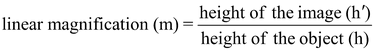# Linear Magnification Produced By Lenses

## LINEAR MAGNIFICATION PRODUCED BY LENSES

The linear magnification produced by a spherical lens (convex or concave) is defined as the ratio of the height of the image (h′) to the height of the object (h). It is a pure ratio and has no units. It is denoted by the letter ‘m’ and is given by,or m = h'/h

The linear magnification (m) is also related to the object distance (u) and image distance (v).

It can be expressed as: m = v/u

⇒ Linear magnification, m = h'/h = v/u

This shows that linear magnification produced by a lens is also equal to the ratio of the image distance (v) to the object distance (u).

### IN CASE OF A CONVEX LENS:

(i) For real and inverted image: According to the New Cartesian Sign Convention, for real and inverted images formed by a convex lens,

Object height (h) is always +ve

Image height (h′) is always –ve

∴ Linear magnification, m = h'/h

m = -ve/+ve or m = -ve

(ii) For virtual and erect image : According to the New Cartesian Sign Convention, for the virtual and erect images formed by a convex lens,

Object height (h) is always +ve

Image height (h′) is always +ve

∴ Linear magnification, m = h'/h

m = +ve/+ve or m = +ve

In case of a convex lens, for real and inverted images, the magnification is always –ve and for the virtual and erect image, the magnification is always +ve.